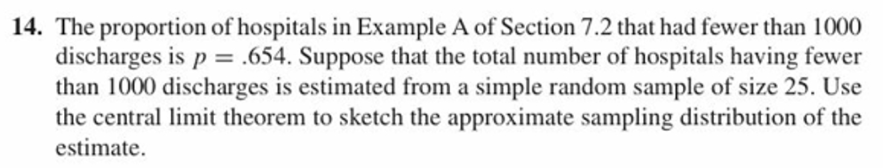# 14. The proportion of hospitals in Example A of Section 7.2 that had fewer than 1000discharges is p = .654. Suppose that the total number of hospitals having fewerthan 1000 discharges is estimated from a simple random sample of size 25. Usethe central limit theorem to sketch the approximate sampling distribution of theestimate.

Questionhelp_outlineImage Transcriptionclose14. The proportion of hospitals in Example A of Section 7.2 that had fewer than 1000 discharges is p = .654. Suppose that the total number of hospitals having fewer than 1000 discharges is estimated from a simple random sample of size 25. Use the central limit theorem to sketch the approximate sampling distribution of the estimate. fullscreen
check_circleExpert Solution
Step 1

Given that the proportion of discharges fewer than 1000 is p = 0.654.

Sample size = 25.

According to central limit theorem the sampling distribution of the estimate will be ...

### Want to see the full answer?

See Solution

#### Want to see this answer and more?

Solutions are written by subject experts who are available 24/7. Questions are typically answered within 1 hour*

See Solution
*Response times may vary by subject and question
Tagged in

### Measures